array_agg函数 array_agg(x) 以数组形式返回x中的所有值。
array_distinct函数 array_distinct(x) 删除数组中重复的元素。
array_except函数 array_except(x, y) 计算两个数组的差集。
array_intersect函数 array_intersect(x, y) 计算两个数组的交集。
array_join函数 array_join(x, delimiter) 使用指定的连接符将数组中的元素拼接为一个字符串。如果数组中包含null元素，则null元素将被忽略。

array_join(x, delimiter, null_replacement) 使用指定的连接符将数组中的元素拼接为一个字符串。如果数组中包含null元素，则null元素将被替换为null_replacement

array_max函数 array_max(x) 获取数组中的最大值。
array_min函数 array_min(x) 获取数组中的最小值。
array_position函数 array_position(x, element) 获取指定元素的下标，下标从1开始。如果指定元素不存在，则返回0。
array_remove函数 array_remove(x, element) 删除数组中指定的元素。
array_sort函数 array_sort(x) 对数组元素进行升序排序。如果有null元素，则null元素排在最后。
array_transpose函数 array_transpose(x) 对矩阵进行转置，即提取二维数组中索引相同的元素组成一个新的二维数组。
array_union函数 array_union(x, y) 计算两个数组的并集。
cardinality函数 cardinality(x) 计算数组中元素的个数。
concat函数 concat(x, y…) 将多个数组拼接为一个数组。
contains函数 contains(x, element) 判断数组中是否包含指定元素。如果包含，则返回true。
element_at函数 element_at(x, y) 返回数组中的第y个元素。
filter函数 filter(x, lambda_expression) 结合Lambda表达式，用于过滤数组中的元素。只返回满足Lambda表达式的元素。
flatten函数 flatten(x) 把将二维数组转换为一维数组。
reduce函数 reduce(x, lambda_expression) 根据Lambda表达式中的定义，对数组中的各个元素进行相加计算，然后返回计算结果。
reverse函数 reverse(x) 对数组中的元素进行反向排列。
sequence函数 sequence(x, y) 通过指定的起始值返回一个数组，其元素为起始值范围内一组连续且递增的值。递增间隔为默认值1。
sequence(x, y, step) 通过指定的起始值返回一个数组，其元素为起始值范围内一组连续且递增的值。自定义递增间隔。
shuffle函数 shuffle(x) 对数组元素进行随机排列。
slice函数 slice(x, start, length) 获取数组的子集。
transform函数 transform(x, lambda_expression) 将Lambda表达式应用到数组的每个元素中。
zip函数 zip(x, y...) 将多个数组合并为一个二维数组，且各个数组中下标相同的元素组成一个新的数组。
zip_with函数 zip_with(x, y, lambda_expression) 根据Lambda表达式中的定义将两个数组合并为一个数组。

## 下标运算符

### 语法

``[x]``

### 参数说明

x 数组下标，从1开始。参数值为bigint类型。

### 示例

• 字段样例
``number:[49,50,45,47,50]``
• 查询和分析语句
``* | SELECT cast(json_parse(number) as array(bigint)) ``
• 查询和分析结果## array_agg函数

array_agg函数会以数组形式返回x中的所有值。

### 语法

``array_agg (x)``

x 参数值为任意数据类型。

array类型。

### 示例

• 查询和分析语句
``* | SELECT array_agg(status) AS array``
• 查询和分析结果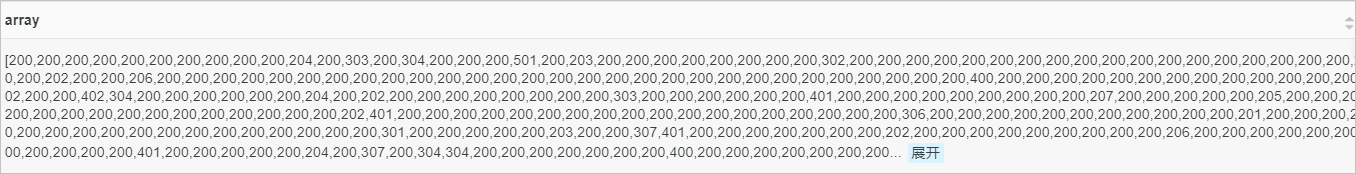## array_distinct函数

array_distinct函数用于删除数组中重复的元素。

### 语法

``array_distinct(x)``

x 参数值为array类型。

array类型。

### 示例

• 字段样例
``number:[49,50,45,47,50]``
• 查询和分析语句
``*| SELECT array_distinct(cast(json_parse(number) as array(bigint)))``
• 查询和分析结果## array_except函数

array_except函数用于计算两个数组的差集。

### 语法

``array_except(x, y)``

x 参数值为array类型。
y 参数值为array类型。

array类型。

### 示例

• 查询和分析语句
``* | SELECT array_except(array[1,2,3,4,5],array[1,3,5,7])``
• 查询和分析结果## array_intersect函数

array_intersect函数用于计算两个数组的交集。

### 语法

``array_intersect(x, y)``

x 参数值为array类型。
y 参数值为array类型。

array类型。

### 示例

• 查询和分析语句
``* | SELECT array_intersect(array[1,2,3,4,5],array[1,3,5,7])``
• 查询和分析结果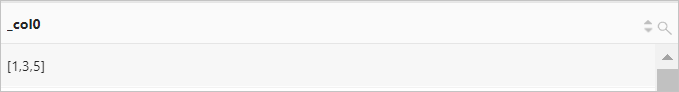## array_join函数

array_join函数使用指定的连接符将数组中的元素拼接为一个字符串。

### 语法

• 使用指定的连接符将数组中的元素拼接为一个字符串。如果数组中包含null元素，则null元素将被忽略。
``array_join(x, delimiter)``
• 使用指定的连接符将数组中的元素拼接为一个字符串。如果数组中包含null元素，则null元素将被替换为null_replacement
``array_join(x, delimiter,null_replacement)``

### 参数说明

x 参数值为任意array类型。
delimiter 连接符，可以为字符串。
null_replacement 用于替换null元素的字符串。

varchar类型。

### 示例

• 查询和分析语句
``* | SELECT array_join(array[null,'Log','Service'],' ','Alicloud')``
• 查询和分析结果## array_max函数

array_max函数用于获取数组中的最大值。

### 语法

``array_max(x) ``

x 参数值为array类型。

### 示例

• 字段样例
``number:[49,50,45,47,50]``
• 查询和分析语句
``*| SELECT array_max(try_cast(json_parse(number) as array(bigint))) AS max_number``
• 查询和分析结果## array_min函数

array_min函数用于获取数组中的最小值。

### 语法

``array_min(x) ``

x 参数值为array类型。

### 示例

• 字段样例
``number:[49,50,45,47,50]``
• 查询和分析语句
``*| SELECT array_min(try_cast(json_parse(number) as array(bigint))) AS min_number``
• 查询和分析结果## array_position函数

array_position函数用于获取指定元素的下标，下标从1开始。如果指定元素不存在，则返回0。

### 语法

``array_position(x, element)``

### 参数说明

x 参数值为数组类型。
element 数组中的一个元素。

bigint类型。

### 示例

• 查询和分析语句
``* | SELECT array_position(array[49,45,47],45)``
• 查询和分析结果## array_remove函数

array_remove函数用于删除数组中指定的元素。

### 语法

``array_remove(x, element)``

### 参数说明

x 参数值为array类型。
element 数组中的一个元素。

array类型。

### 示例

• 查询和分析语句
``* | SELECT array_remove(array[49,45,47],45)``
• 查询和分析结果## array_sort函数

array_sort函数用于对数组元素进行升序排序。如果有null元素，则null元素排在最后。

### 语法

``array_sort(x)``

x 参数值为array类型。

array类型。

### 示例

• 查询和分析语句
``* | SELECT array_sort(array['b','d',null,'c','a'])``
• 查询和分析结果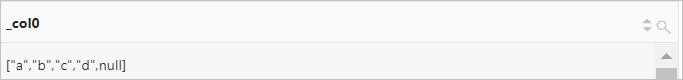## array_transpose函数

array_transpose函数用于对矩阵进行转置，即提取二维数组中索引相同的元素组成一个新的二维数组。

### 语法

``array_transpose(x)``

### 参数说明

x 参数值为array(double)类型。

array(double)类型。

### 示例

• 查询和分析语句
``* | SELECT array_transpose(array[array[0,1,2,3],array[10,19,18,17],array[9,8,7]])``
• 查询和分析结果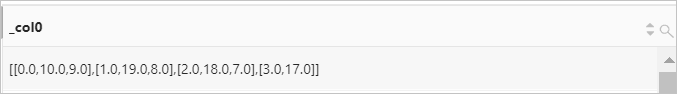## array_union函数

array_union函数用于计算两个数组的并集。

### 语法

``array_union(x, y)``

x 参数值为array类型。
y 参数值为array类型。

array类型。

### 示例

• 查询和分析语句
``* | SELECT array_union(array[1,2,3,4,5],array[1,3,5,7])``
• 查询和分析结果## cardinality函数

cardinality函数用于计算数组中元素的个数。

### 语法

``cardinality(x)``

x 参数值为array类型。

bigint类型。

### 示例

• 字段样例
``number:[49,50,45,47,50]``
• 查询和分析语句
``*| SELECT cardinality(cast(json_parse(number) as array(bigint)))``
• 查询和分析结果## concat函数

concat函数用于将多个数组拼接为一个数组。

### 语法

``concat(x, y…)``

x 参数值为array类型。
y 参数值为array类型。

array类型。

### 示例

• 查询和分析语句
``* | SELECT concat(array['red','blue'],array['yellow','green'])``
• 查询和分析结果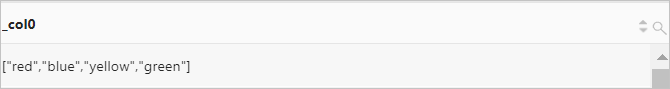## contains函数

contains函数用于判断数组中是否包含指定元素。如果包含，则返回true。

### 语法

``contains(x, element)``

### 参数说明

x 参数值为数组类型。
element 数组中的一个元素。

boolean类型。

### 示例

• 字段样例
``region:["cn-hangzhou","cn-shanghai","cn-beijing"]``
• 查询和分析语句
``*| SELECT contains(cast(json_parse(region) as array(varchar)),'cn-beijing')``
• 查询和分析结果## element_at函数

element_at函数用于返回数组中的第y个元素。

### 语法

``element_at(x, y)``

### 参数说明

x 参数值为array类型。
y 数组下标，从1开始。参数值为bigint类型。

### 示例

• 字段样例
``number:[49,50,45,47,50]``
• 查询和分析语句
``````* |
SELECT
element_at(cast(json_parse(number) AS array(varchar)), 2)``````
• 查询和分析结果## filter函数

filter函数和Lambda表达式结合，用于过滤数组中的元素。只返回满足Lambda表达式的元素。

### 语法

``filter(x, lambda_expression)``

### 参数说明

x 参数值为array类型。
lambda_expression Lambda表达式。更多信息，请参见Lambda表达式

array类型。

### 示例

• 查询和分析语句
``* | SELECT filter(array[5,-6,null,7],x -> x > 0)``
• 查询和分析结果## flatten函数

flatten函数用于将二维数组转换为一维数组。

### 语法

``flatten(x)``

x 参数值为array类型。

array类型。

### 示例

• 查询和分析语句
``* | SELECT flatten(array[array[1,2,3,4],array[5,2,2,4]])``
• 查询和分析结果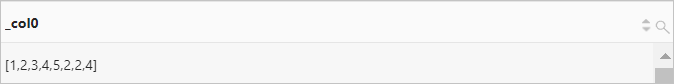## reduce函数

reduce函数将根据Lambda表达式中的定义，对数组中的各个元素进行相加计算，然后返回计算结果。

### 语法

``reduce(x, lambda_expression)``

### 参数说明

x 参数值为array类型。
lambda_expression Lambda表达式。更多信息，请参见Lambda表达式

bigint类型。

### 示例

• 查询和分析语句
``* | SELECT reduce(array[5,20,50],0,(s, x) -> s + x, s -> s)``
• 查询和分析结果## reverse函数

reverse函数用于对数组中的元素进行反向排列。

### 语法

``reverse(x)``

x 参数值为array类型。

array类型。

### 示例

• 查询和分析语句
``* | SELECT reverse(array[1,2,3,4,5])``
• 查询和分析结果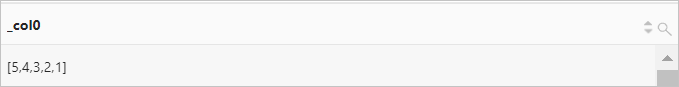## sequence函数

sequence函数通过指定的起始值返回一个数组，其元素为起始值范围内一组连续且递增的值。

### 语法

• 递增间隔为默认值1。
``sequence(x, y)``
• 自定义递增间隔。
``sequence(x, y, step)``

### 参数说明

x 参数值为bigint类型、timestamp类型（Unix时间戳、日期和时间表达式）。
y 参数值为bigint类型、timestamp类型（Unix时间戳、日期和时间表达式）。
step 数值间隔。

• `interval ' n' year to month`，表示间隔为n年。
• `interval 'n' day to second`，表示间隔为n天。

array类型。

### 示例

• 示例1：返回0~10之间的偶数。
• 查询和分析语句
``* | SELECT sequence(0,10,2)``
• 查询和分析结果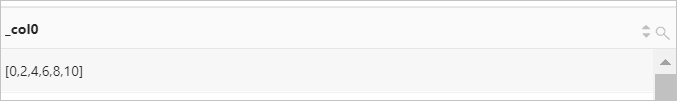• 示例2：返回2017-10-23到2021-08-12之间的日期，间隔为1年。
• 查询和分析语句
``ww* | SELECT  sequence(from_unixtime(1508737026),from_unixtime(1628734085),interval '1' year to month )``
• 查询和分析结果• 示例3：返回1628733298,1628734085之间的Unix时间戳，间隔为60秒。
• 查询和分析语句
``* | SELECT  sequence(1628733298,1628734085,60)``
• 查询和分析结果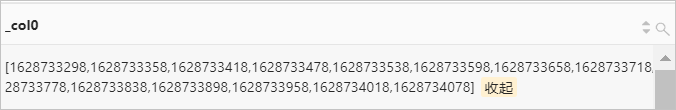## shuffle函数

shuffle函数用于对数组元素进行随机排列。

### 语法

``shuffle(x)``

x 参数值为array类型。

array类型。

### 示例

• 查询和分析语句
``*| SELECT shuffle(array[1,2,3,4,5])``
• 查询和分析结果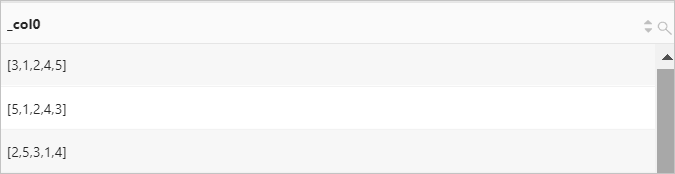## slice函数

slice函数用于返回数组的子集。

### 语法

``slice(x, start, length)``

### 参数说明

x 参数值为array类型。
start 指定索引开始的位置。
• 如果start为负数，则从末尾开始。
• 如果start为正数，则从头部开始。
length 指定子集中元素的个数。

array类型。

### 示例

• 查询和分析语句
``* | SELECT slice(array[1,2,4,5,6,7,7],3,2)``
• 查询和分析结果## transform函数

transform函数用于将Lambda表达式应用到数组的每个元素中。

### 语法

``transform(x, lambda_expression)``

### 参数说明

x 参数值为array类型。
lambda_expression Lambda表达式。更多信息，请参见Lambda表达式

array类型。

### 示例

• 查询和分析语句
``* | SELECT transform(array[5,6],x -> x + 1)``
• 查询和分析结果## zip函数

zip函数用于将多个数组合并为一个二维数组，且各个数组中下标相同的元素组成一个新的数组。

### 语法

``zip(x, y...) ``

x 参数值为array类型。
y 参数值为array类型。

array类型。

### 示例

• 查询和分析语句
``* | SELECT zip(array[1,2,3], array['1b',null,'3b'],array[1,2,3])``
• 查询和分析结果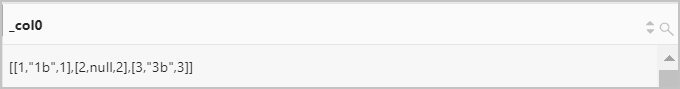## zip_with函数

zip_with函数将根据Lambda表达式中的定义将两个数组合并为一个数组。

### 语法

``zip_with(x, y, lambda_expression)``

### 参数说明

x 参数值为array类型。
y 参数值为array类型。
lambda_expression Lambda表达式。更多信息，请参见Lambda表达式

array类型。

### 示例

• 查询和分析语句
``SELECT zip_with(array[1,2], array[3,4],(x,y) -> x + y)``
• 查询和分析结果